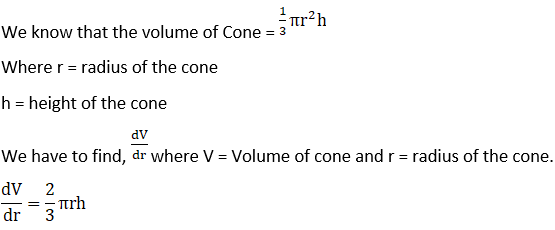# RD Sharma Solutions For Class 12 Maths Exercise 13.1 Chapter 13 Derivative as a Rate Measurer

RD Sharma Solutions for Class 12 Maths Exercise 13.1 Chapter 13 Derivative as a Rate Measurer is provided here. The first exercise of Chapter 13 pertains to the topic of finding the derivative of a given function as its rate measurer. These solutions are formulated by BYJU’S experts in an interactive manner to create interest in learning among students. It also provides explanatory diagrams and tables for comparative study, which makes it easy to learn the crucial topics.

Students who find difficulty in solving these topics are suggested to go through RD Sharma Solutions for Class 12 Maths Exercise 13.1 of Chapter 13. These solutions will help them to a great extent.

## RD Sharma Solutions Class 12 Chapter 13 Derivative as a Rate Measurer Exercise 13.1: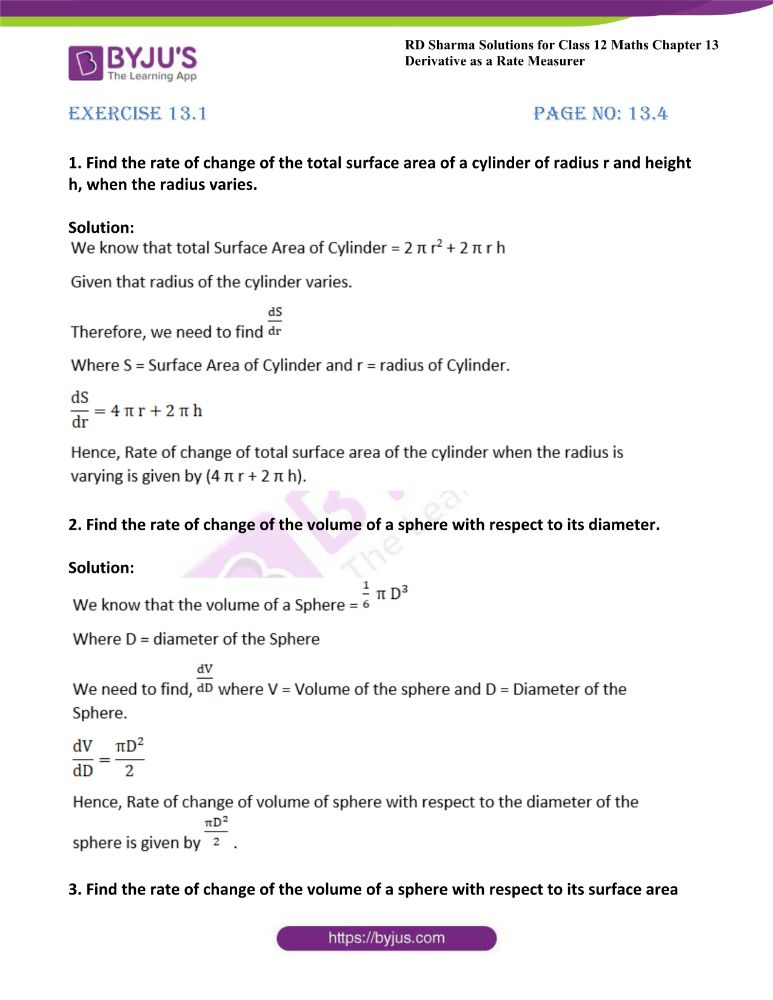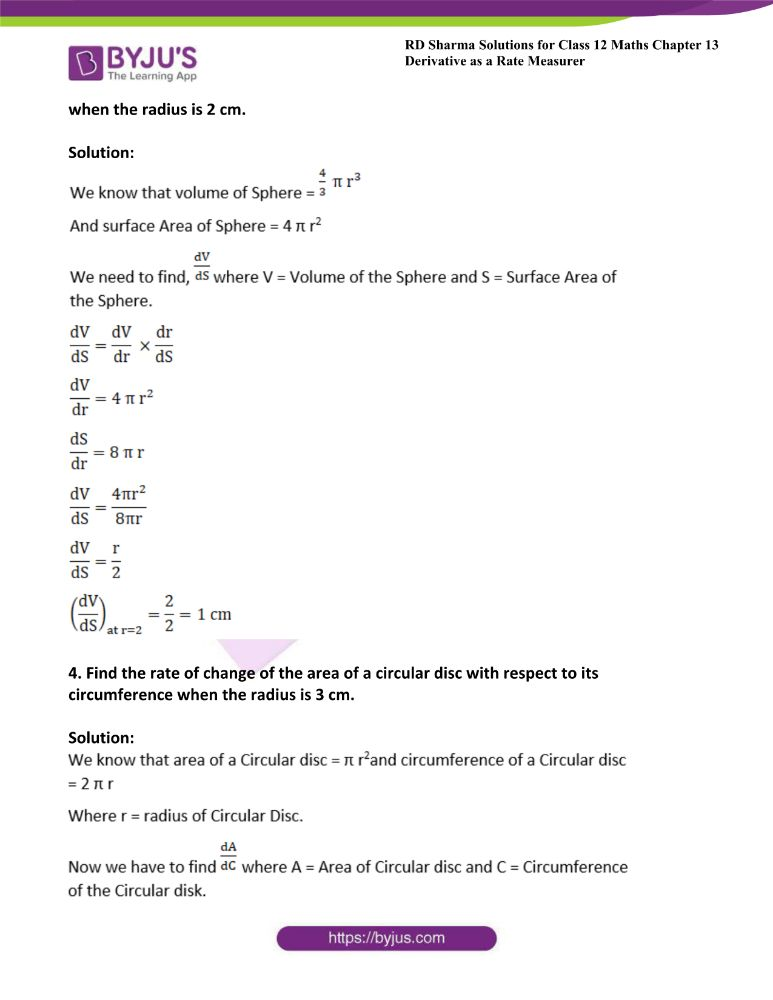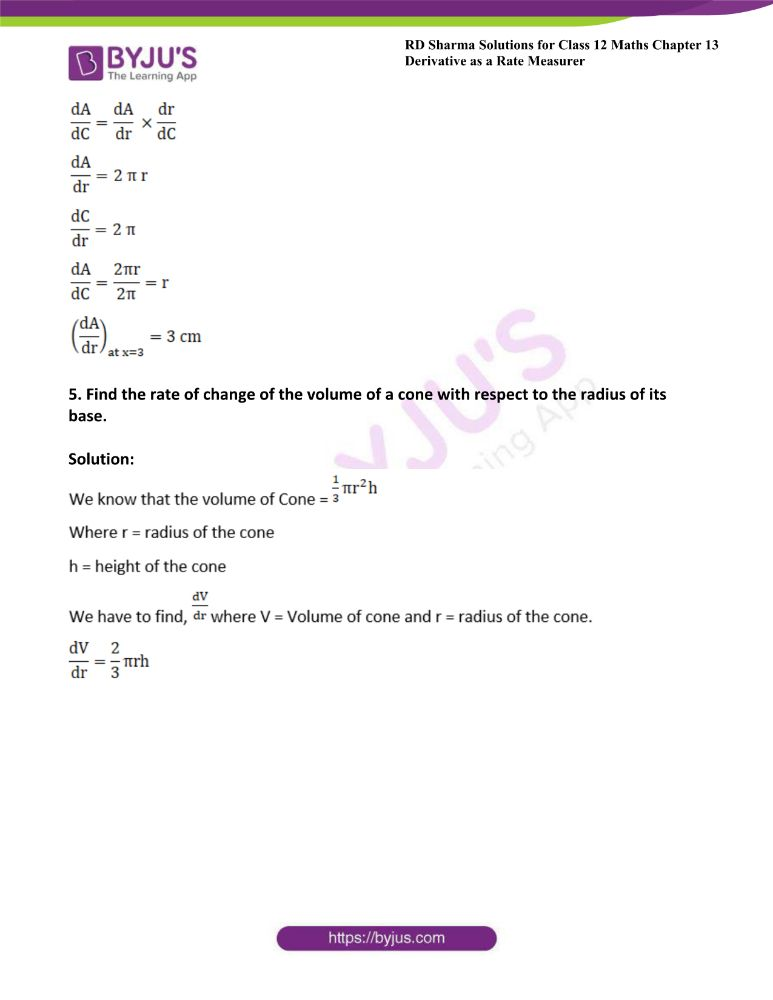### Access another exercise of RD Sharma Solutions For Class 12 Chapter 13 – Derivative as a Rate Measurer

Exercise 13.2 Solutions

### Access answers to Maths RD Sharma Solutions For Class 12 Chapter 13 – Derivative as a Rate Measurer Exercise 13.1

Page No: 13.4

1. Find the rate of change of the total surface area of a cylinder of radius r and height h, when the radius varies.

Solution: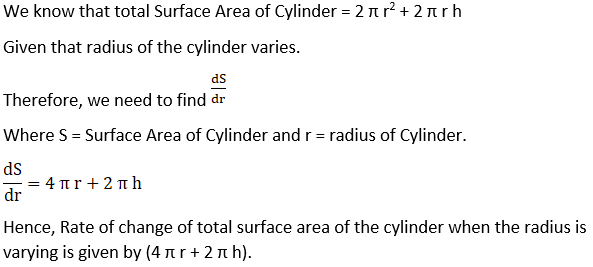2. Find the rate of change of the volume of a sphere with respect to its diameter.

Solution: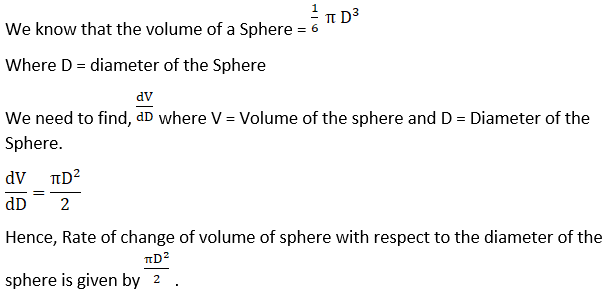3. Find the rate of change of the volume of a sphere with respect to its surface area when the radius is 2 cm.

Solution: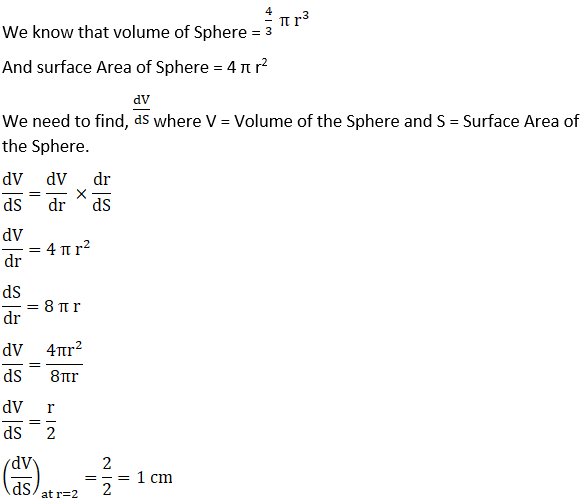4. Find the rate of change of the area of a circular disc with respect to its circumference when the radius is 3 cm.

Solution: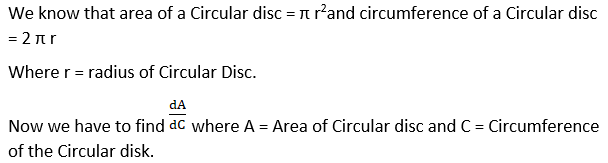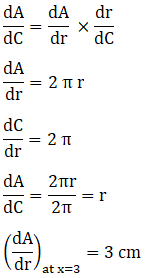5. Find the rate of change of the volume of a cone with respect to the radius of its base.

Solution: